## UNIT 4 PROGRAMMING IN C++ LANGUAGE PDF FREE DOWNLOAD INTERVIEW, EXAMPLES

### Statements:-

Statements are the instructions given to the Computer to perform any kind of action.

### Null Statement:-

A null statement is useful in those case where syntax of the language requires the presence of a statement but logic of program does not give permission to do anything then we can use null statement. A null statement is nothing only a ;. A null (or empty statement have the following form:

; // only a semicolon (;)

### Compound Statement :-

A compound statement is a group of statements enclosed in the braces { }. A Compound statement is useful in those case where syntax of the language requires the presence of only one statement but logic of program have to do more thing i.e., we want to give more than one statement in place of one statement then we can use compound statement.

{
St-1;
St-2;
:
:
}

### Statement Flow Control:-

In a program , statements may be executed sequentially, selectively, or iteratively. Every programming language provides three constructs:

1. Sequence Constructs
2. Selection Constructs
3. Iteration Constructs

#### Sequence Construct:-

The sequence construct means the statements are being executed sequentially. It represents the default flow of statements.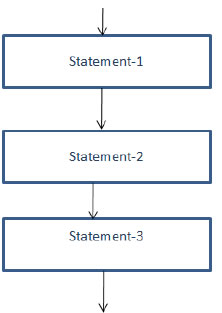#### Looping or Iteration Statements:-

Looping the iteration construct means repetition of set of statements depending upon a condition test. The iteration statements allow a set of instructions to be performed repeatedly until a certain condition is true.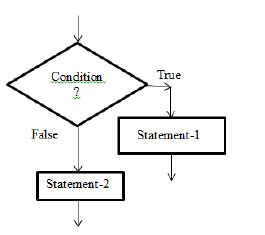#### Looping or Iteration Statements:-

Looping the iteration construct means repetition of set of statements depending upon a condition test. The iteration statements allow a set of instructions to be performed repeatedly until a certain condition is true.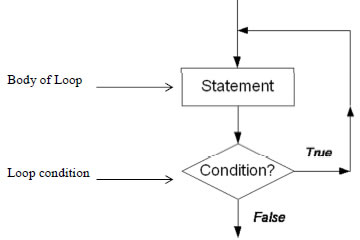#### There are two types of loops:-

##### 1. Entry-controlled loop :-

In entry-controlled loop first of all loop condition is checked and then body of loop is executed is condition is true. If loop condition is false in the starting the body of loop is not executed even once.

##### 2. Exit-controlled loop :-

In exit-controlled loop first body of loop is executed once and then loop condition is checked. If condition is true then the body of loop will be executed again. It means in this type of loop, loop body will be executed once without checking loop condition. Selection

##### Statements :-

There are two types of selection statements in C++ :
1. if statement
2. switch statement

##### 1. if Statement :

If statement have three forms (a) if … else Statement :- It is useable, when we have to performs an action if a condition is True and we have to perform a different action if the condition is false. The syntax of if…else statement is: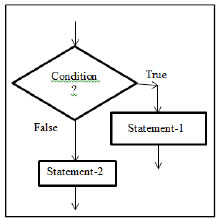if ( < conditional expression > )
{
< statement-1 or block-1>;
// statements to be executed when conditional expression is true.
}
else
{
< statement-2 or block-2>;
// statements to be executed when conditional expression is false.
}

If the is evaluated to true then the < statement-1 or block-1> (statement under if ( ) block ) will be executed otherwise the (statements under else block) would be executed. if there exists only one program statement under if( ) block then we may omit curly braces { }.

For example, a program to check whether a given number is greater than 100 or not is given below:

#include <iostream.h>
void main()
{
int x;
cout<< “\nEnter a number: “;
cin>> x;
if( x > 100 )
cout<< “That number is greater than 100\n”;
else
cout<< “That number is not greater than 100\n”;
In this program if the conditional expression (x>100) in the if statement is true, the program prints one message; if it isn’t, it prints the other. Here’s output from two different invocations of the program: Enter a number: 300 That number is greater than 100 Enter a number: 3 That number is not greater than 100}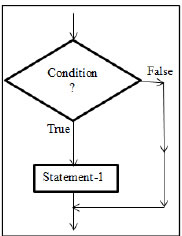#### (b) Simple if statement:-

The else part in if … else statement is optional, if we omit the else part then it becomes simple if statement. This statement is usable, when we have to either perform an action if a condition is True or skips the action if the condition is false. The syntax of simple if statement is:

if ( < conditional expression > )
{
< statement-1 or block-1>;
// statements to be executed when conditional expression is true.
}

Here will be executed only if evaluates true. if there exists only one program statement under if( ) block then we may omit curly braces { }. eg.,

#include <iostream.h>
void main()
{
int x;
cout<< “Enter a number: “;
cin>> x;
if( x > 100 )
cout<< “That number is greater than 100\n”;
}

In the above example, program’s output when the number entered by the user is greater than 100: Enter a number: 2000

That number is greater than 100

If the number entered is not greater than 100, the program will terminate without printing the second line.

#### (c) The if-else-if ladder :-

This statement allows you to test a number of mutually exclusive cases and only execute one set of statements for which condition evaluates true first. The syntax is:

if ( <condition -1> )
statement-1; // do something if condition-1 is satisfied (True)
else if ( <condition – 2 >)
statement-3 ; // do something if condition -2 is satisfied (True)
else if (<condition – 3 >)
statement-3 ; // do something if condition- 3 is satisfied (True)
:
: // many more n-1 else - if ladder may come
:
else if( < condition – n >)
statement-n ; // do something if condition – n is satisfied (True)
else
statement-m ; // at last do here something when none of the
// above conditions gets satisfied (True)
}

<> in syntax is known as a place holder, it is not a part of syntax, do not type it while writing program. It only signifies that anything being kept there varies from program to program. [ ] is also not a part of syntax , it is used to mark optional part of syntax i.e. all part of syntax between [ ] is optional.

In the above syntax there are ladder of multiple conditions presented by each if( ) , all of these conditions are mutually exclusive. If one of them would evaluates true then the statement followed that condition will be executed and all the conditions below it would not be evaluated (checked).

Say suppose if condition-3 gets satisfy (i.e. evaluates true value for the condition), then statement- 3 gets executed and all other conditions below it would be discarded.

If none of the n if ( ) conditions gets satisfied then the last else part always gets executed. It is not compulsory to add an else at the last of the ladder.

We can also write a group of statement enclosed in curly braces { } (as a compound statement) in place of any statement ( statement-1. Statement-2,……., statement-n) if required in above syntax. For example, a program which accept number of week’s day (1-7) and print is equivalent name of week day ( Monday for 1,……, Sunday for 7). using the if-else-if ladder is given below:

#include< iostream.h>
#include<conio.h>
void main()
{
int day;
cout<<“ Enter a number (between 1 and 7):”<< endl;
cin>>day;
if (day= =1)
cout<<“ Monday”<< endl;
else if (day= =2)
cout<<“ Tuesday”<<endl;
else if( day= =3)
cout<<“ Wednesday”<<endl;
else if(day= =4)
cout<<“ Thursday”<<endl;
else if(day= =5)
cout<<“ Friday”<< endl;
else if(day= =6)
cout<<“ Saturday”<< endl;
else if(day= =7)
cout<<“ Sunday”<< endl;
else
cout<<“ You enter a wrong number”<< endl;
getch();
}

Nested if Statement:- If an if statement is written in the if or else clause of another if statement then it is known as nested if. Some possible syntax of nested if statements given below: Syntax 1:-

if ( <outer- condition > )
{
if ( <inner-condition> )
{
//some statements to be executed
// on satisfaction of inner if ( ) condition.
} // end of scope of inner if( )
//some statements to be executed
// on satisfaction of outer if ( ) condition.
} // end of the scope of outer if( )

Syntax 2:-
if ( <outer- condition > )
{
if ( <inner-condition> )
{
//some statements to be executed
// on satisfaction of inner if ( ) condition.
}
else
{
// statements on failure of inner if( )
}
//some statements to be executed
// on satisfaction of outer if ( ) condition.
}
else
{
// statements on failure of outer if( )
}

### NCERT Books Free Pdf Download for Class 5, 6, 7, 8, 9, 10 , 11, 12 Hindi and English Medium

 Mathematics Biology Psychology Chemistry English Economics Sociology Hindi Business Studies Geography Science Political Science Statistics Physics Accountancy